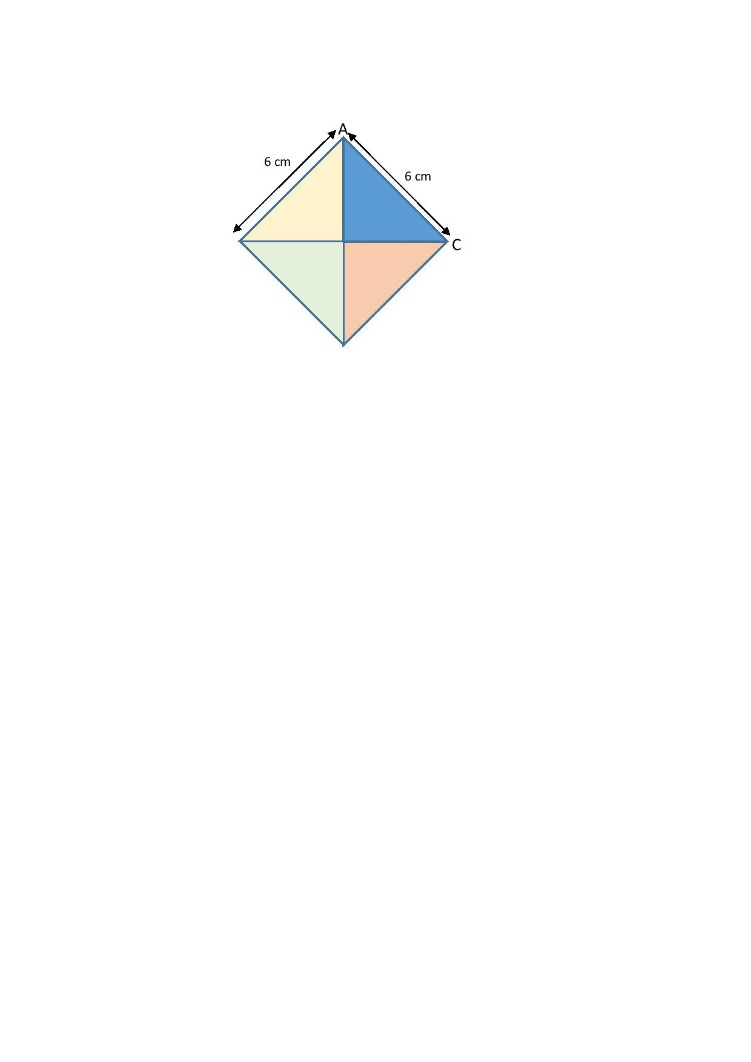# AnswerThe triangle ABC is one quarter of a square of side 6 cm.

Area of triangle ABC = 1/4 × 6 × 6 = 9

Let AB = BC = R, the radius of the circle.

Then Area of triangle ABC = 1/2 × R × R = 1/2 R² = 9

R² = 18

Area of 3/4 of the circle = 3/4 × π × R² = 3/4 × 3.14 × 18 = 42.39

Area of the figure = 9 + 42.39 = 51.39 cm²

0 Replies 0 Likes ✔Accepted Answer